# 大数据渲染的渐进式方案## 二、初识fps

``````<!DOCTYPE html>
<html lang="en">
<meta charset="UTF-8" />
<meta http-equiv="X-UA-Compatible" content="IE=edge" />
<meta name="viewport" content="width=device-width, initial-scale=1.0" />
<title>Document</title>
<style>
.box {
width: 100px;
height: 100px;
background: red;
animation: normal-animate 5s linear infinite;
margin: 50px 0;
position: absolute;
}

@keyframes normal-animate {
0% {
left: 0px;
}
50% {
left: 100px;
}
100% {
left: 0px;
}
}
</style>
<body>
<h1>时间切片案例</h1>
<p>
<span>input事件测试</span>
<input />
</p>
<button onclick="sync()">同步</button>
<div class="box"></div>
<script>
let index = 0;
const sync = () => {
let now = performance.now(); /* 开始时间*/
while (performance.now() - now <= 5000) {
// 必须执行5秒种，且是同步
index++;
}
};
</script>
</body>
</html>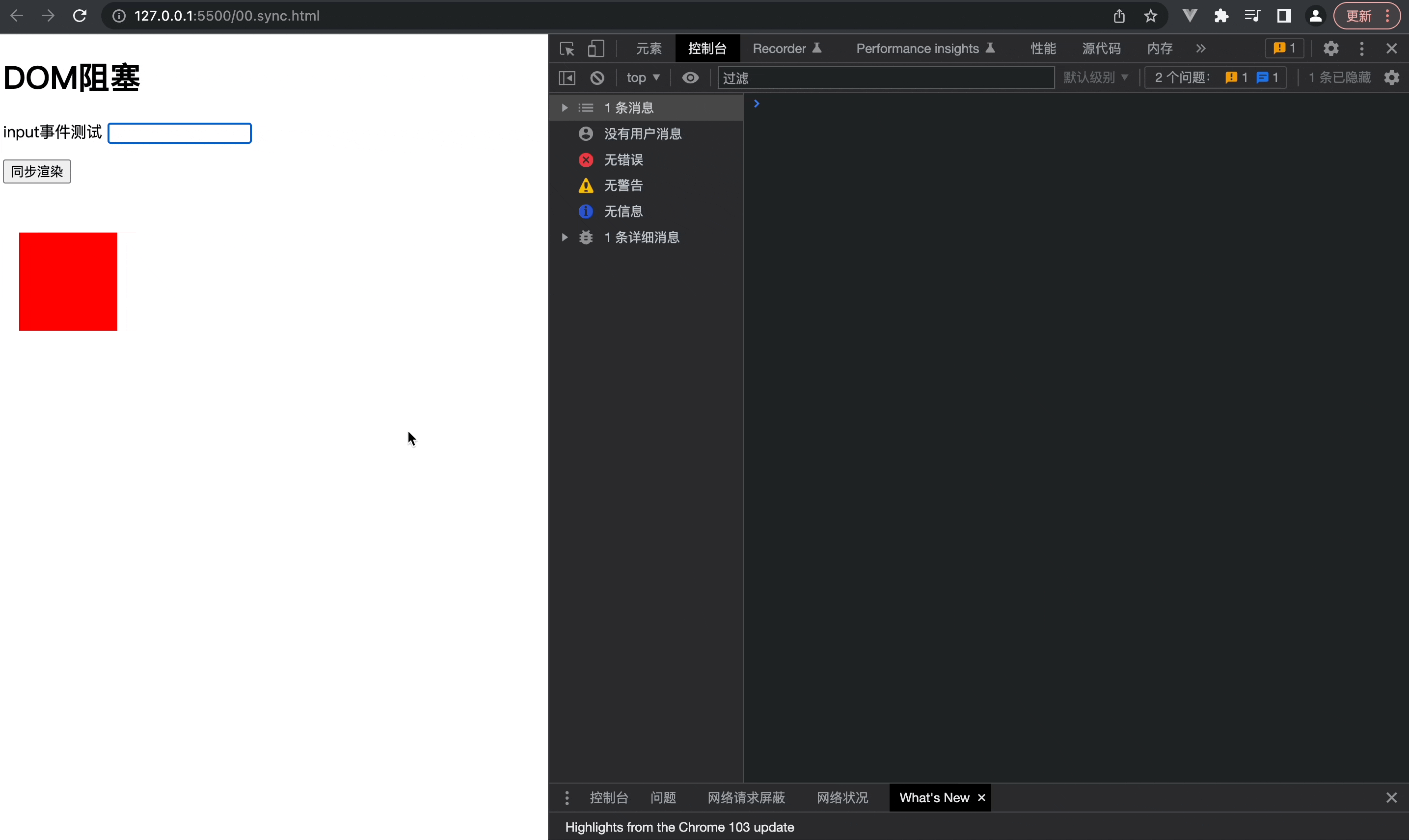## 三、纤细的时光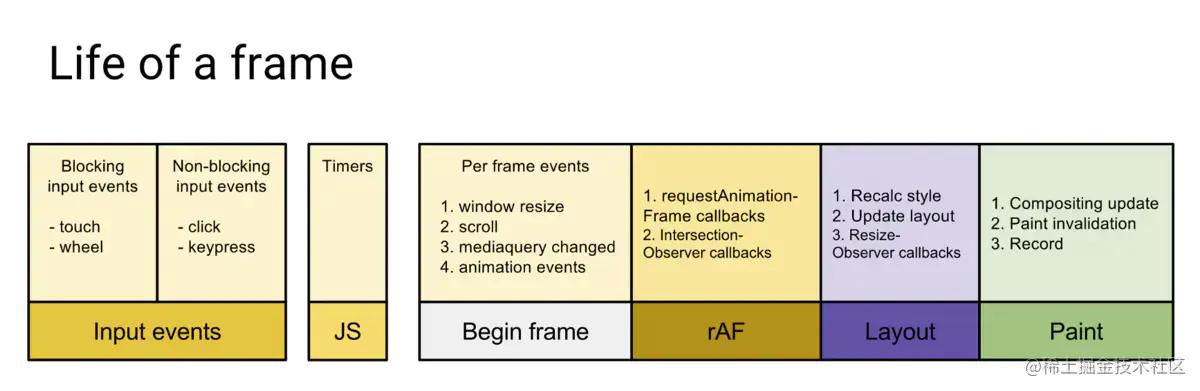1. 事件处理/event
2. js脚本执行
3. scroll/resize 事件
4. requestAnimationFrame
5. 布局/绘制

`温馨提示：在接下来的案例中，为了节省空间，保持更好阅读体验，永远都以第一个案例中的单页html为蓝本，只展示增量代码，不展示全量代码`

``````let index = 0;
const RAF = () => {
let now = performance.now(); /* 开始时间*/
const task = (handle) => {
console.log("执行")
index++
if (performance.now() - now <= 5000) {
}else{
console.log(`在5秒内执行了\${index}次，本次实验帧率为\${ index/5 }fps`);
}
};
};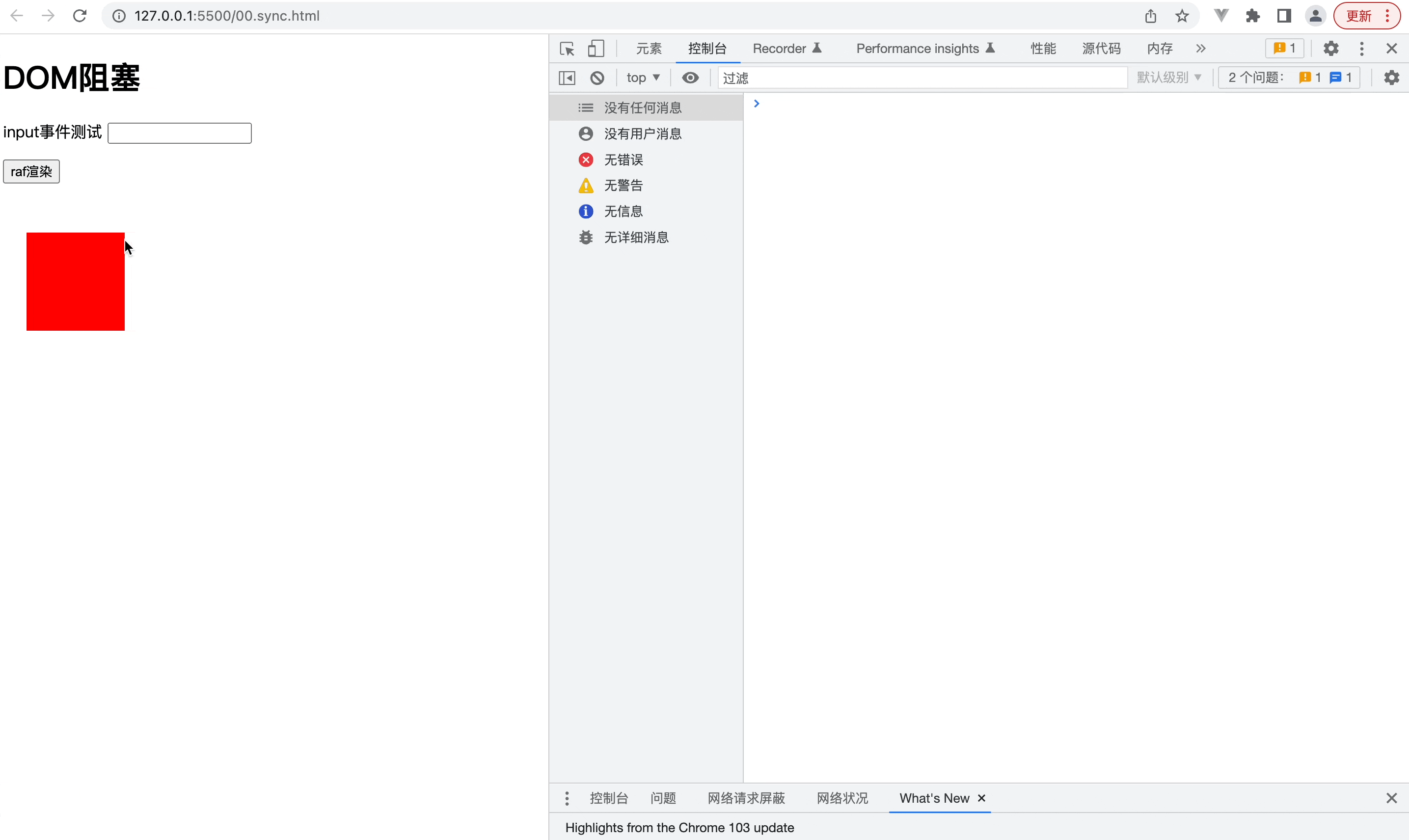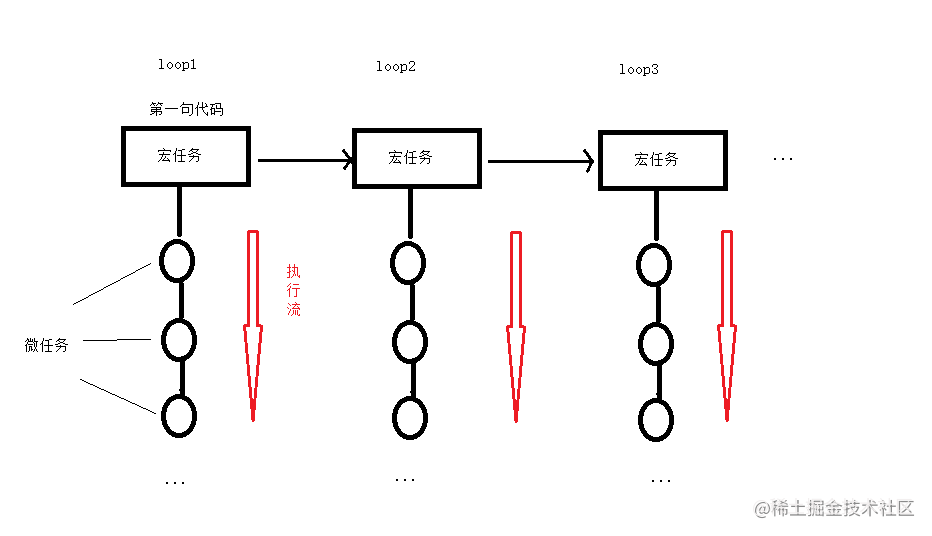``````let index = 0;
const setTimeFn = () => {
let now = performance.now(); /* 开始时间*/
const task = (handle) => {
console.log("执行")
index++
if (performance.now() - now <= 5000) {
}else{
console.log(`在5秒内执行了\${index}次，比RAF的300次要\${ index > 300 ? "多":"少" }`);
}
};
};
// 只是单纯的替换了宏任务，其他逻辑不变；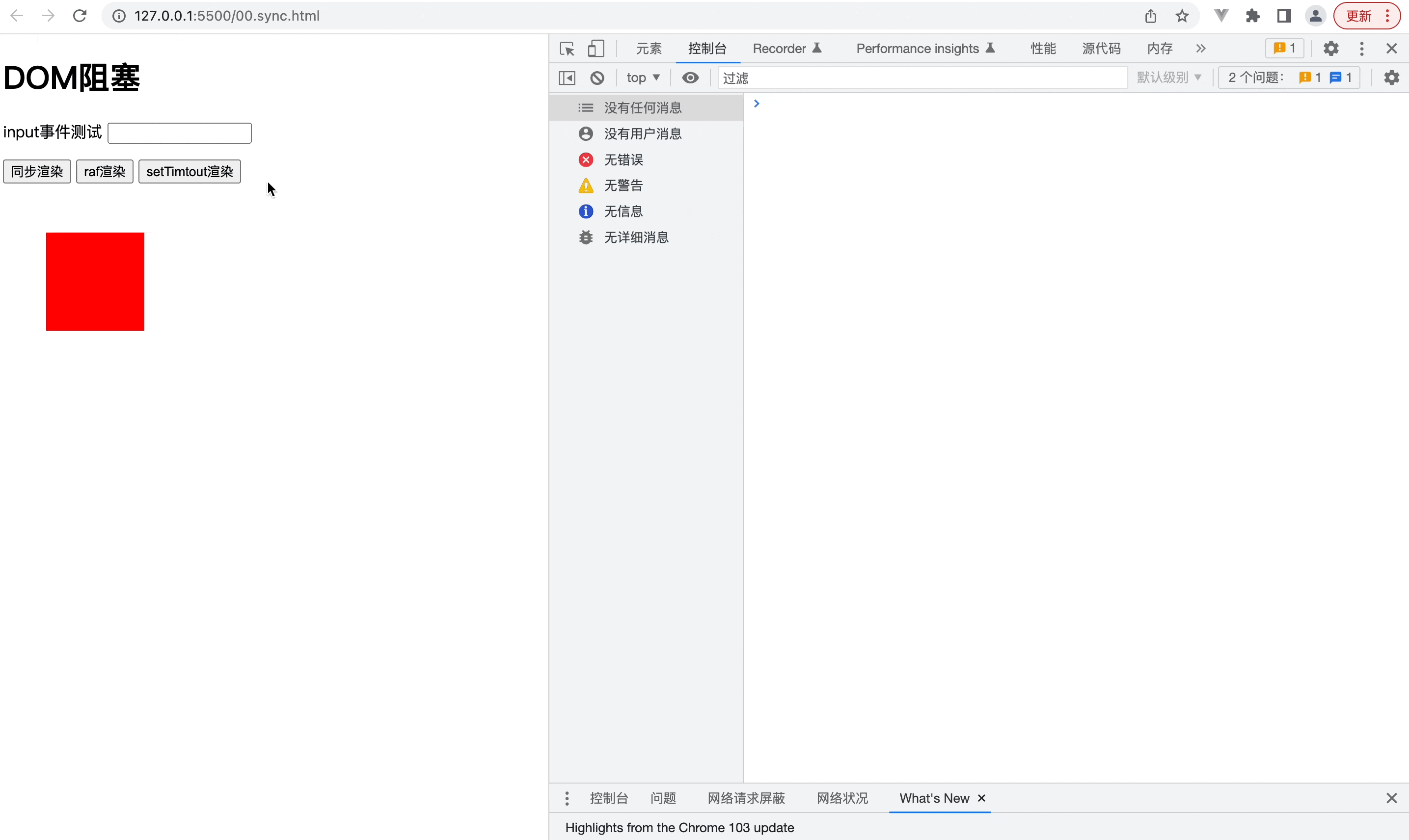我这边测试的结果是setTimeout消费的函数次数是requestAnimationFrame的3倍还多一点；因此我们可以分析一下：

## 五、案例实践

``````<!DOCTYPE html>
<html lang="en">
<meta charset="UTF-8" />
<meta http-equiv="X-UA-Compatible" content="IE=edge" />
<meta name="viewport" content="width=device-width, initial-scale=1.0" />
<title>Document</title>
<style>
.circle {
width: 10px;
height: 10px;
position: absolute;
}
</style>
<body>
<button onclick="render()">render</button>
<div id="root"></div>
<script>
const factorArr = [0,1,2,3,4,5,6,7,8,9,'a','b','c','d','e','f']
/* 颜色 */
function getColor() {
const r = factorArr[Math.floor(Math.random() * 16)];
const g = factorArr[Math.floor(Math.random() * 16)];
const b = factorArr[Math.floor(Math.random() * 16)];
return `#\${r}\${g}\${b}`;
}
/* 位置 */
function getPostion(position) {
const { width, height } = position;
return {
left: Math.ceil(Math.random() * width) + "px",
top: Math.ceil(Math.random() * height) + "px",
};
}
/* 色块组件 */
function Circle(position) {
return {
background: getColor(),
...getPostion(position),
};
}

const root = document.getElementById("root");

function renderRoot(num) {
const fragment = document.createElement("fragment");
for (let index = 0; index < num; index++) {
const div = document.createElement("div");
const { left, top, background } = Circle({
width: 1000,
height: 600,
});
div.className = "circle";
div.style.left = left;
div.style.top = top;
div.style.backgroundColor = background;
fragment.appendChild(div);
}

root.appendChild(fragment);
}

const render = () => {
renderRoot(100000);
};
</script>
</body>
</html>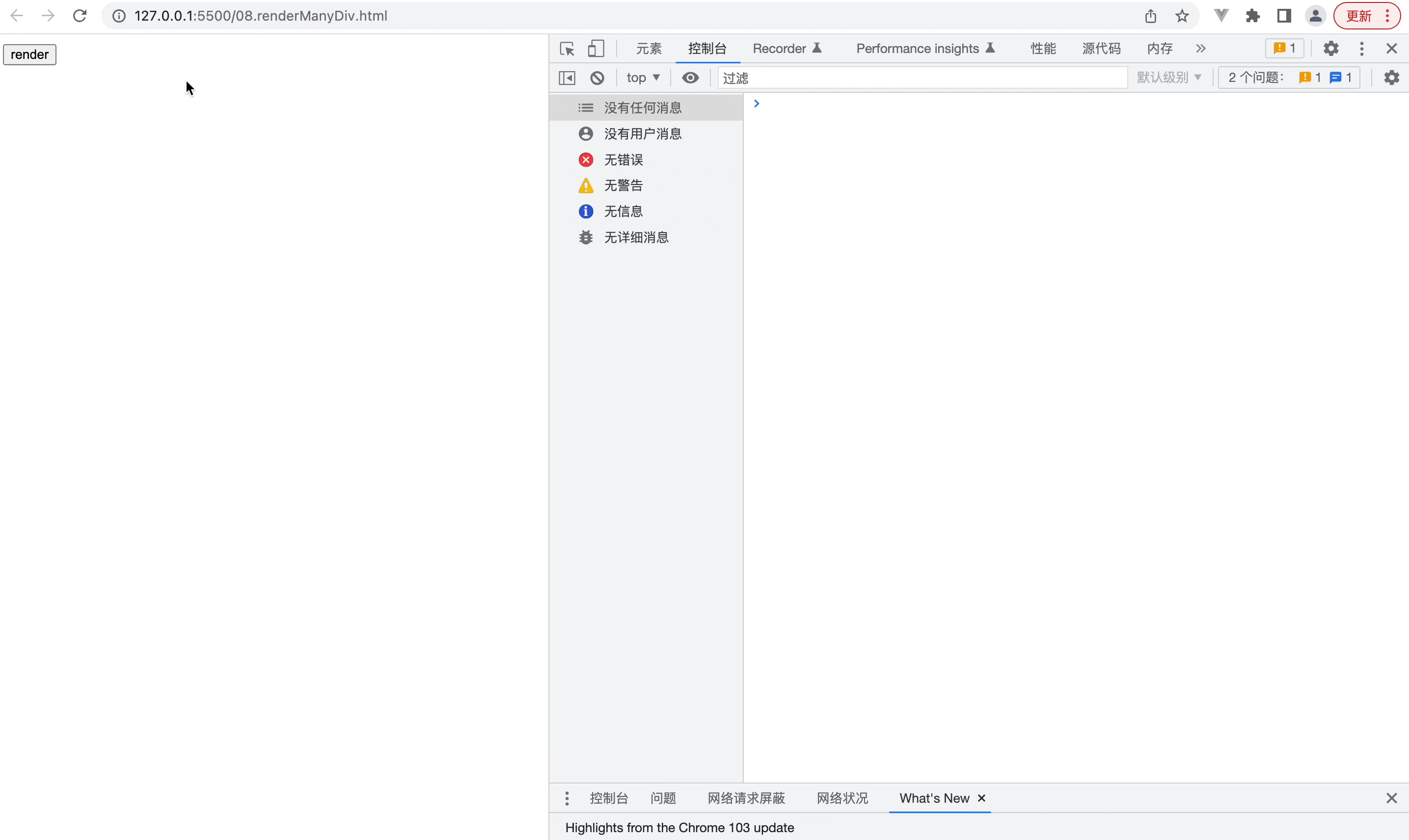## 五、帧渲染

``````// 提示：在上面html中需要新增一个按钮调用renderSlice

const scheduler = (size, scale, index) => {
let start = performance.now(); /* 开始时间*/
const handleTask = () => {
renderRoot(size);
console.log(index, scale);
if (index < scale) {
index++;
}else {
let end = performance.now(); /* 结束时间*/
console.log(`\${(end - start) / 1000}s`);
}
};
};

const renderSlice = () => {
const size = 2000;
const scale = 500000 / size;
scheduler(size, scale, 0);
};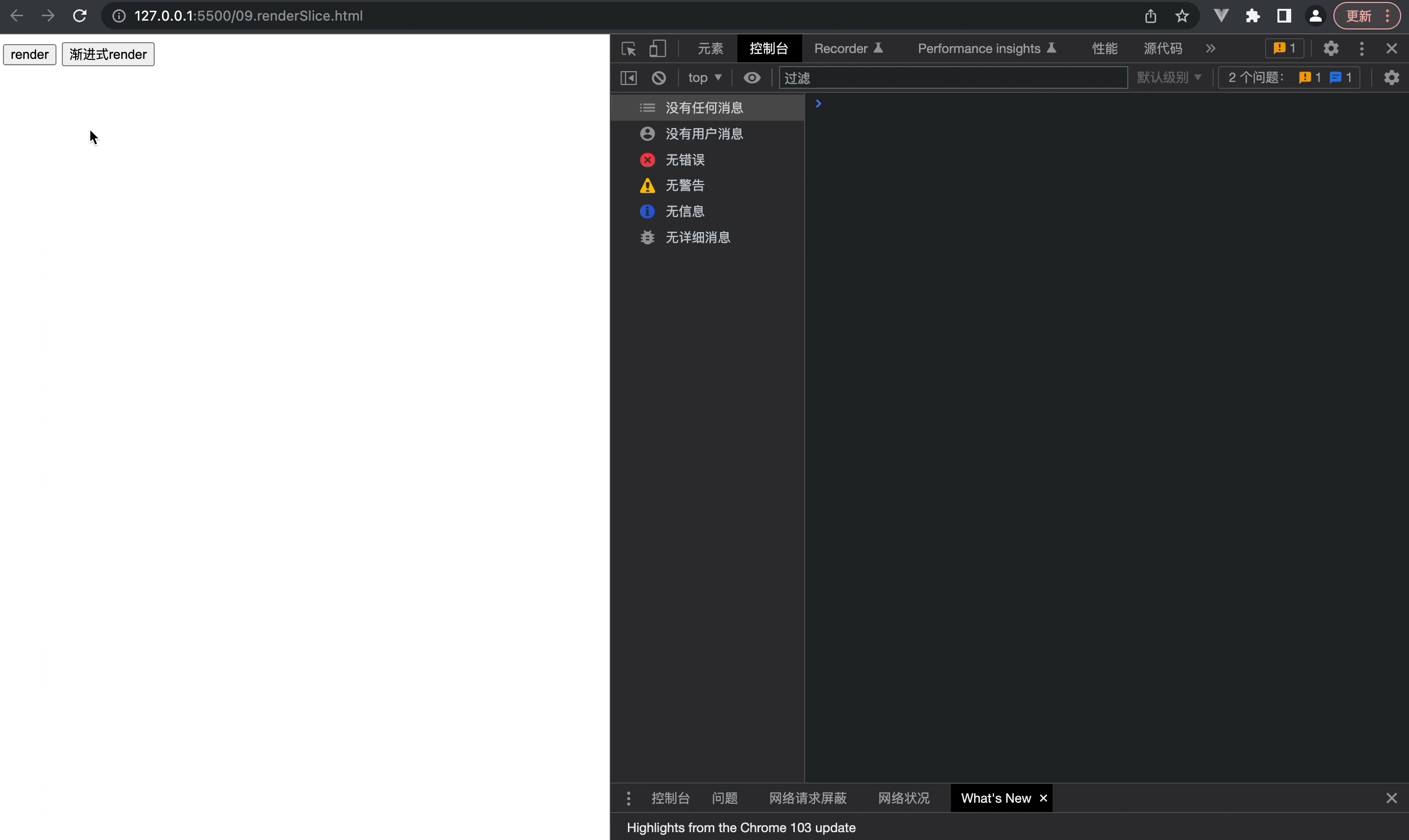``````// 提示：在上面html中需要新增一个按钮调用renderSlice

const scheduler = (size, scale, index) => {
let start = performance.now(); /* 开始时间*/
const handleTask = () => {
renderRoot(size);
console.log(index, scale);
if (index < scale) {
index++;
}else {
let end = performance.now(); /* 结束时间*/
console.log(`\${(end - start) / 1000}s`);
}
};
};

const renderSlice = () => {
const size = 1000;
const scale = 500000 / size;
scheduler(size, scale, 0);
};## 六、宏渲染

``````const scheduler = (size, scale, index) => {
let start = performance.now(); /* 开始时间*/
const handleTask = () => {
renderRoot(size);
console.log(index, scale);
if (index < scale) {
index++;
}else {
let end = performance.now(); /* 结束时间*/
console.log(`\${(end - start) / 1000}s`);
}
};
};

const renderSlice = () => {
const size = 1000;
const scale = 500000 / size;
scheduler(size, scale, 0);
};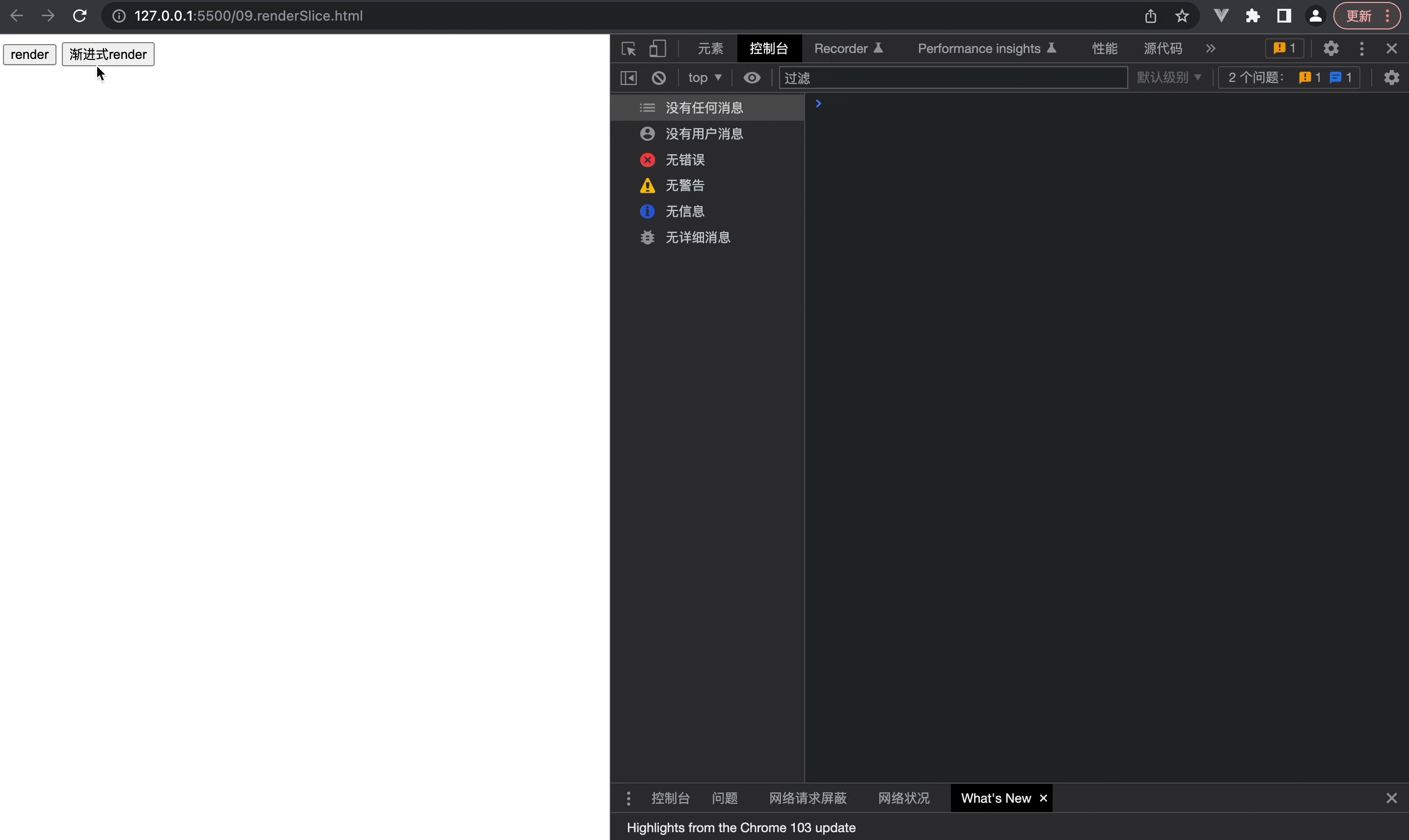奇怪，感觉好想也没有好多少；多做几次的实验结果是平均就少了1s，虽然是优化了一些，但是远低于我们的预期，这是为什么呢？

``````const async = (size, scale, index) => {
let start = performance.now(); /* 开始时间*/
const { port1, port2 } = new MessageChannel();
port2.onmessage = (e) => {
renderRoot(size);
if (index < scale) {
index++;
port1.postMessage("去给我执行任务去");
}else {
let end = performance.now(); /* 结束时间*/
console.log(`\${(end - start) / 1000}s`);
}
};

port1.postMessage("去给我执行任务去");
};

const renderSlice = () => {
const size = 1000;
const scale = 100000 / size;
async(size, scale, 0);
};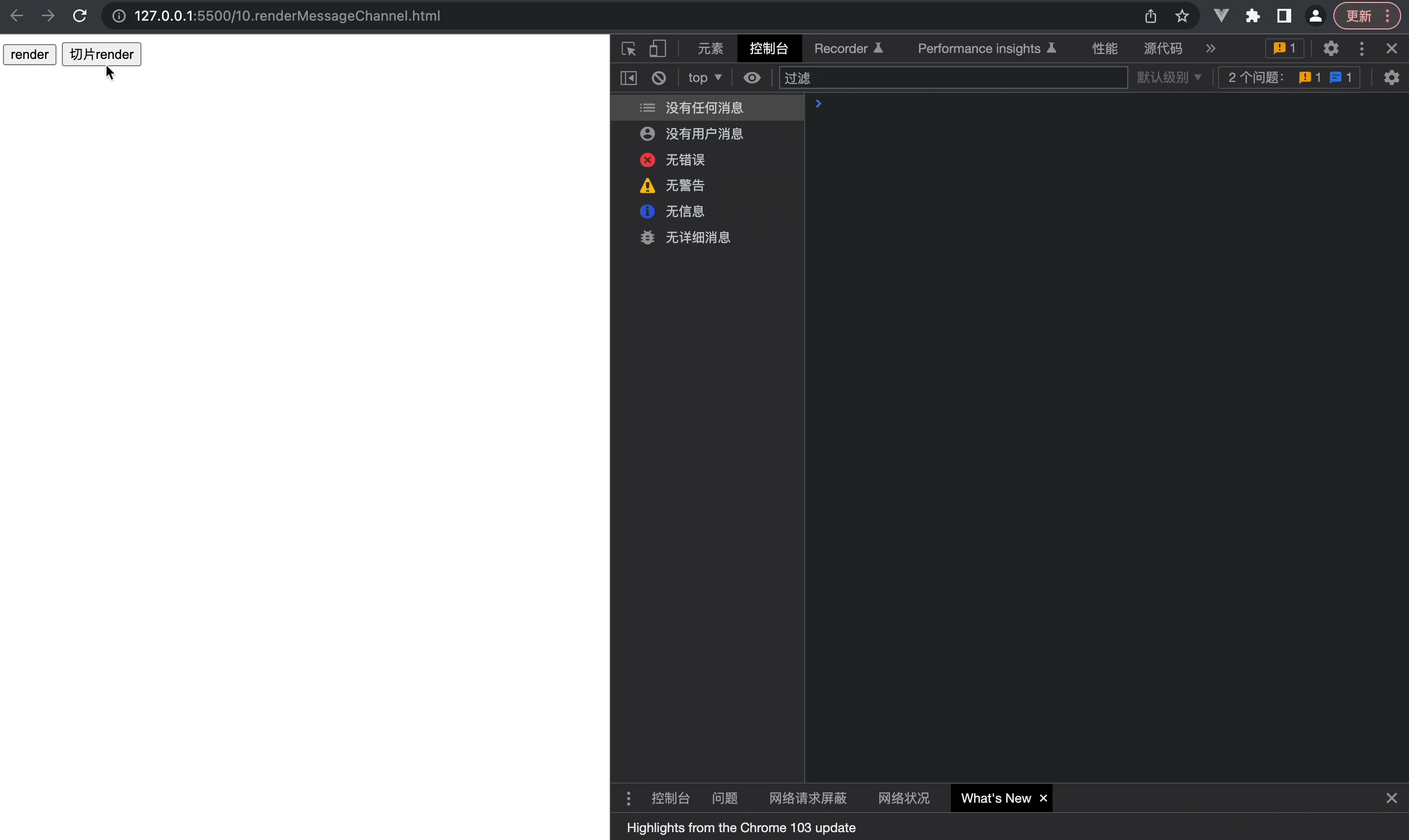可以看到，快了一倍不止，所以我们可以总结，MessageChannel，拥有更高的效率，兼容性也好，并且属于一个宏任务，可以作为我们目前这个阶段的最佳方案；

## 七、结合react

``````const { port1, port2 } = new MessageChannel();

port2.onmessage = (e) => {
console.log(`MessageChannel`);
};

setTimeout(() => {
console.log("setTimeout");
});

port1.postMessage("");

requestAnimationFrame(() => {
console.log("requestAnimationFrame");
});

Promise.resolve().then(() => {
console.log("Promise");
});

// 输出的顺序